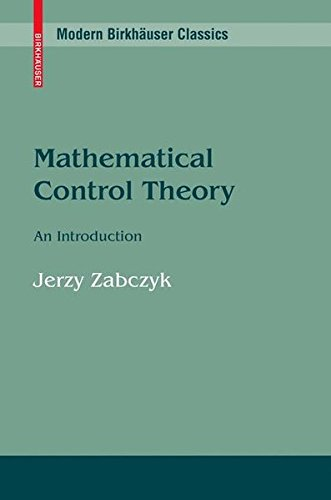# Mathematical Control Theory: An Introduction (Systems & Control: Foundations & Applications)Type
Book
Authors
ISBN 10
0817636455
ISBN 13
9780817636456
Category
Unknown  [ Browse Items ]
Publication Year
1992
Publisher
Pages
260
Abstract

Description
"This book is designed as a graduate text on the mathematical theory of deterministic control. It covers a remarkable number of topics... The book includes material on the realization of both linear and nonlinear systems, impulsive control, and positive linear systems—subjects not usually covered in an 'introductory' book... To get so much material in such a short space, the pace of the presentation is brisk. However, the exposition is excellent, and the book is a joy to read. A novel one-semester course covering both linear and nonlinear systems could be given... The book is an excellent one for introducing a mathematician to control theory. The book presents a large amount of material very well, and its use is highly recommended."   —Bulletin of the AMS Mathematical Control Theory: An Introduction presents, in a mathematically precise manner, a unified introduction to deterministic control theory. With the exception of a few more advanced concepts required for the final part of the book, this presentation requires only a knowledge of basic facts from linear algebra, differential equations, and calculus. In addition to classical concepts and ideas, the author covers the stabilization of nonlinear systems using topological methods, realization theory for nonlinear systems, impulsive control and positive systems, the control of rigid bodies, the stabilization of infinite dimensional systems, and the solution of minimum energy problems. The book will be ideal for a beginning graduate course in mathematical control theory, or for self study by professionals needing a complete picture of the mathematical theory that underlies the applications of control theory. - from Amzon
Biblio Notes

Number of Copies